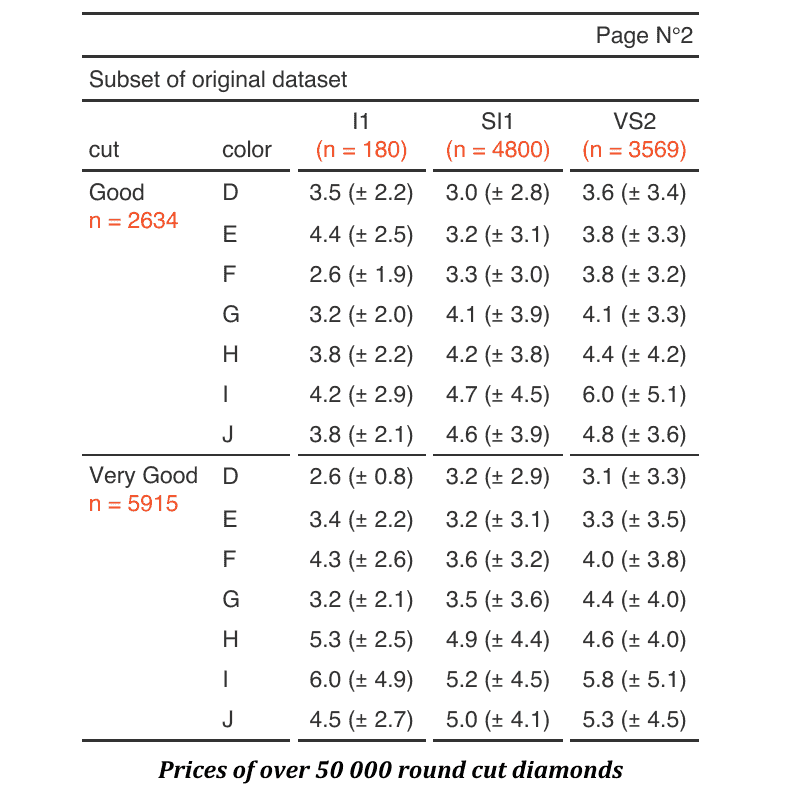It tabulates a data.frame representing an aggregation which is then transformed as a flextable with as_flextable. The function allows to define any display with the syntax of flextable in a table whose layout is showing dimensions of the aggregation across rows and columns.## Usage

tabulator(
x,
rows,
columns,
datasup_first = NULL,
datasup_last = NULL,
hidden_data = NULL,
row_compose = list(),
...
)

# S3 method for tabulator
summary(object, ...)

## Arguments

x

an aggregated data.frame

rows

column names to use in rows dimensions

columns

column names to use in columns dimensions

datasup_first

additional data that will be merged with table and placed after the columns presenting the row dimensions.

datasup_last

additional data that will be merged with table and placed at the end of the table.

hidden_data

additional data that will be merged with table, the columns are not presented but can be used with compose() or mk_par() function.

row_compose

a list of call to as_paragraph() - these calls will be applied to the row dimensions (the name is used to target the displayed column).

...

named arguments calling function as_paragraph(). The names are used as labels and the values are evaluated when the flextable is created.

object

an object returned by function tabulator().

## Value

an object of class tabulator.

## Methods (by generic)

• summary(tabulator): call summary() to get a data.frame describing mappings between variables and their names in the flextable. This data.frame contains a column named col_keys where are stored the names that can be used for further selections.

## Note

This is very first version of the function; be aware it can evolve or change.

as_flextable.tabulator(), summarizor(), as_grouped_data(), tabulator_colnames()

## Examples

if (FALSE) {
set_flextable_defaults(digits = 2, border.color = "gray")

library(data.table)
# example 1 ----
if (require("stats")) {
dat <- aggregate(breaks ~ wool + tension,
data = warpbreaks, mean
)

cft_1 <- tabulator(
x = dat, rows = "wool",
columns = "tension",
mean = as_paragraph(as_chunk(breaks)),
(N) = as_paragraph(as_chunk(length(breaks), formatter = fmt_int))
)

ft_1 <- as_flextable(cft_1)
ft_1
}

# example 2 ----
if (require("ggplot2")) {
multi_fun <- function(x) {
list(mean = mean(x), sd = sd(x))
}

dat <- as.data.table(ggplot2::diamonds)
dat <- dat[cut %in% c("Fair", "Good", "Very Good")]

dat <- dat[, unlist(lapply(.SD, multi_fun),
recursive = FALSE
),
.SDcols = c("z", "y"),
by = c("cut", "color")
]

tab_2 <- tabulator(
x = dat, rows = "color",
columns = "cut",
z stats = as_paragraph(as_chunk(fmt_avg_dev(z.mean, z.sd, digit2 = 2))),
y stats = as_paragraph(as_chunk(fmt_avg_dev(y.mean, y.sd, digit2 = 2)))
)
ft_2 <- as_flextable(tab_2)
ft_2 <- autofit(x = ft_2, add_w = .05)
ft_2
}

# example 3 ----
# data.table version
dat <- melt(as.data.table(iris),
id.vars = "Species",
variable.name = "name", value.name = "value"
)
dat <- dat[,
list(
avg = mean(value, na.rm = TRUE),
sd = sd(value, na.rm = TRUE)
),
by = c("Species", "name")
]
# dplyr version
# library(dplyr)
# dat <- iris %>%
#   pivot_longer(cols = -c(Species)) %>%
#   group_by(Species, name) %>%
#   summarise(avg = mean(value, na.rm = TRUE),
#   sd = sd(value, na.rm = TRUE),
#   .groups = "drop")

tab_3 <- tabulator(
x = dat, rows = c("Species"),
columns = "name",
mean (sd) = as_paragraph(
as_chunk(avg),
" (", as_chunk(sd), ")"
)
)
ft_3 <- as_flextable(tab_3)
ft_3

init_flextable_defaults()
}